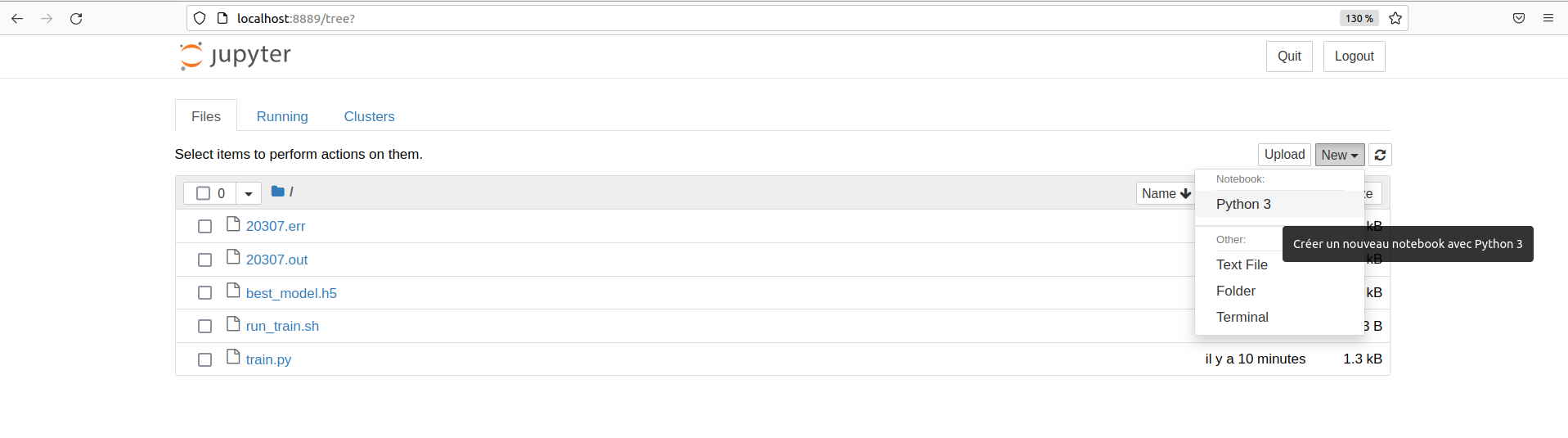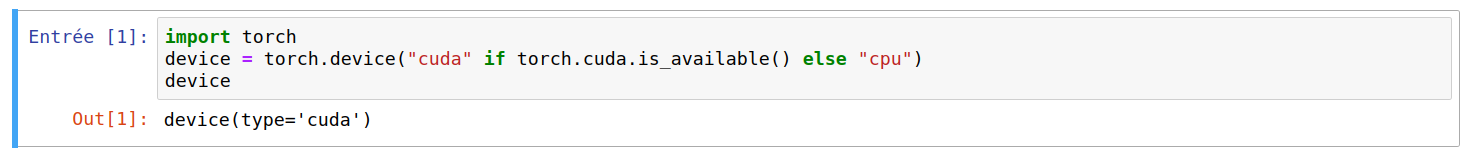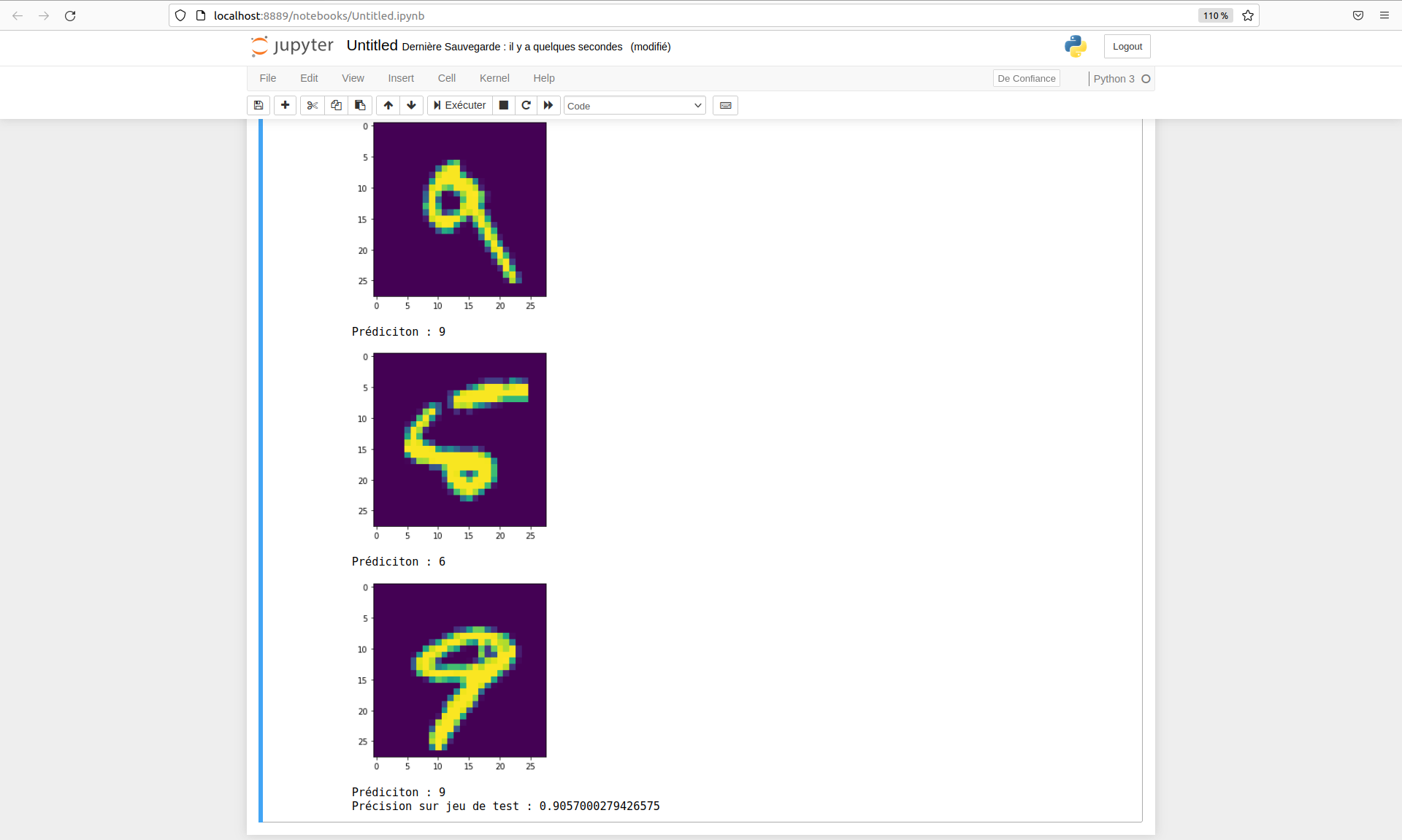# Running an AI algorithm on GPUs

## Introduction

This tutorial aims to run an AI algorithm in python on computational clusters. It is composed of two parts:

• launching the training of an AI model on the Bigfoot cluster using a submission script.
• launch a Jupyter Notebook to load the previously trained model and run it with an interactive job

## Prerequisites

• Have a local ssh configuration with transparent proxy.

``````Linux bigfoot 5.10.0-14-amd64 #1 SMP Debian 5.10.113-1 (2022-04-29) x86_64
Welcome to Bigfoot cluster!

:            :
.'            :
_.-"              :
_.-"                  '.
..__...____...-"                       :
: \_\                                    :
:    .--"                                 :
`.__/  .-" _                               :
/  /  ," ,-                            .'
(_)(`,(_,'L_,_____       ____....__   _.'
"' "             """""""          """

GPU, GPU, GPU, ... ;-)

Type 'chandler' to get cluster status
Type 'recap.py' to get cluster properties

Sample OAR submissions:
# Get a A100 GPU and all associated cpu and memory resources:
oarsub -l /nodes=1/gpu=1 --project test -p "gpumodel='A100'" "nvidia-smi -L"
# Get a MIG partition of an A100 on a devel node, to make some tests
oarsub -l /nodes=1/gpu=1/migdevice=1 --project test -t devel "nvidia-smi -L"

Last login: Fri Jul 22 15:38:45 2022 from 129.88.178.43
``````

## Launching a training

In this part, the goal is to launch the training of an artificial intelligence model on the Bigfoot computing cluster via a submission script.

### Python environment

First of all, you have to choose your working environment, which must include Python and the necessary modules. For the management of Python modules we will use conda.

The following script is used to source conda:

``````login@bigfoot:~\$ source /applis/environments/conda.sh
``````

Then you just have to choose one of the conda environments available on Bigfoot.

The following command displays the different environments available:

``````login@bigfoot:~\$ conda env list
# conda environments:
#
base                  *  /applis/common/miniconda3
GPU                      /applis/common/miniconda3/envs/GPU
f-ced-gpu                /applis/common/miniconda3/envs/f-ced-gpu
fidle                    /applis/common/miniconda3/envs/fidle
fidle-orig               /applis/common/miniconda3/envs/fidle-orig
gpu_preprod              /applis/common/miniconda3/envs/gpu_preprod
julia                    /applis/common/miniconda3/envs/julia
tensorflow1.x_py3_cuda10.1     /applis/common/miniconda3/envs/tensorflow1.x_py3_cuda10.1
tensorflow2.x_py3_cuda10     /applis/common/miniconda3/envs/tensorflow2.x_py3_cuda10
torch1.x_py3_cuda10      /applis/common/miniconda3/envs/torch1.x_py3_cuda10
torch1.x_py3_cuda92      /applis/common/miniconda3/envs/torch1.x_py3_cuda92
``````

The following command displays all the modules available in a given environment.

``````login@bigfoot:~\$ conda list -n GPU
``````

### Training program

In this example, we will build a basic classification network of the MNIST DataSet and train it.

Let’s start by moving to our dedicated directory on the Bettik storage service by replacing login with your Perseus ID in the following command:

``````login@bigfoot:~\$ cd /bettik/login
``````

To create the classification network, we need to create a train.py file in a tuto_ia/ directory containing the following code:

``````import tensorflow as tf

from tensorflow.keras.datasets import mnist
from tensorflow.keras.utils import to_categorical

from tensorflow.keras import Input
from tensorflow.keras.layers import Dense, Activation
from tensorflow.keras.models import Model

from tensorflow.python.client import device_lib
print("GPUs Available: ", tf.config.experimental.list_physical_devices('GPU'))

(X_train, y_train), (X_test, y_test) = mnist.load_data()
num_train  = X_train.shape
img_height = X_train.shape
img_width  = X_train.shape
X_train = X_train.reshape((num_train, img_width * img_height))
y_train = to_categorical(y_train, num_classes=10)

num_classes = 10
xi      = Input(shape=(img_height*img_width,))
xo      = Dense(num_classes)(xi)
yo      = Activation('softmax')(xo)
model   = Model(inputs=[xi], outputs=[yo])

model.compile(loss='categorical_crossentropy',
metrics=['accuracy'])

callbacks = [tf.keras.callbacks.ModelCheckpoint(
filepath="best_model.h5",
monitor='val_accuracy',
mode='max',
save_best_only=True)]

model.fit(X_train, y_train,
batch_size=128,
epochs=20,
verbose=1,
validation_split=0.1,
callbacks=callbacks)
``````
``````import torch
import torch.nn as nn
from torchvision import datasets, transforms
from tqdm import tqdm
import sys

class Classifier(nn.Module):
def __init__(self):
super(Classifier, self).__init__()
self.linear = nn.Linear(in_features=28*28, out_features=10)
self.activation = nn.Softmax(dim=1)

def forward(self, x):
x = self.linear(x)
output = self.activation(x)
return output

prev_acc = 0
for epoch in range(epochs):
print(f"Epoch {epoch+1}/{epochs}")
model.train()
X, Y = batch
X, Y = X.to(device), Y.to(device)
Y_pred = model(X)
loss = nn.CrossEntropyLoss()(Y_pred, Y)
loss.backward()
optimizer.step()
model.eval()
accuracy = 0
X, Y = X.to(device), Y.to(device)
y_pred = model(X)
y_classes = torch.argmax(y_pred, dim=1)
accuracy += torch.sum(y_classes == Y)
print(f"Validation accuracy : {accuracy}")
if accuracy > prev_acc:
torch.save(model, "best_model.pt")
prev_acc = accuracy

def main():
use_cuda = torch.cuda.is_available()
device = torch.device("cuda" if use_cuda else "cpu")
print(f"Used device : {device}")

transform=transforms.Compose([
transforms.ToTensor(),
torch.squeeze,
torch.flatten
])

train_size = int(0.8 * len(dataset))
val_size = len(dataset) - train_size
train_dataset, val_dataset = torch.utils.data.random_split(dataset, [train_size, val_size])

model = Classifier().to(device)

if __name__ == "__main__":
main()
``````

This small python program creates the classification model, trains it on a training dataset, display its progress on the standard output, and saves the best model in the tuto_ia/ directory.

### Write an execution script

Once the training program is ready, you have to write a script to submit the launch of a job on the computing machines.

To do this, simply create a run_train.sh file containing the following code, and replace login by your Perseus ID and your-project by your project name:

``````#!/bin/bash

#OAR -n tuto_training
#OAR -l /nodes=1/gpu=1,walltime=0:30:00
#OAR --stdout %jobid%.out
#OAR --stderr %jobid%.err
#OAR --project your-project
#OAR -p gpumodel='V100'

source /applis/environments/cuda_env.sh bigfoot 10.2
source /applis/environments/conda.sh
conda activate GPU

python train.py
``````

This script allows you to prepare the OAR request by specifying the resources needed, the project concerned, or the management of standard inputs and outputs.

It also prepares the necessary conda and cuda environments, and runs the model training.

### Submit the job

We can now launch the job on the computing machines.

First, we have to make the script executable with the following command :

``````login@bigfoot:/bettik/login/tuto_ia\$ chmod +x run_train.sh
``````

We can then submit its execution via the resource manager with the command :

``````login@bigfoot:/bettik/login/tuto_ia\$ oarsub -S ./run_train.sh
[ADMISSION RULE] Modify resource description with type constraints
OAR_JOB_ID=18545
``````

The job has been submitted and an ID has been assigned to it: OAR_JOB_ID=18545

### Follow the execution of the job

We can now follow the progress of our job using a few commands by replacing login by your Perseus ID :

``````login@bigfoot:/bettik/login/tuto_ia\$ oarstat -u login
Job id    S User     Duration   System message
--------- - -------- ---------- ------------------------------------------------
18545     W login       0:00:00 R=32,W=0:30:0,J=B,N=Entrainement_tuto,P=your-project (Karma=0.014,quota_ok)
``````

We can see that the job is waiting for resources, in fact its status is W for Waiting. You will have to wait until the requested resource is available.

If we try again later, we obtain :

``````login@bigfoot:/bettik/login/tuto_ia\$ oarstat -u login
Job id    S User     Duration   System message
--------- - -------- ---------- ------------------------------------------------
18545     R login       0:00:13 R=32,W=0:30:0,J=B,N=Entrainement_tuto,P=your-project (Karma=0.014,quota_ok)
``````

We see that the job has been running for 13 seconds, its status is R for Running.

The model is now trained, and it is saved in the tuto_ia/ directory.

## Exploiting the model

In this part, the goal is now to use an interactive job in order to launch a Jupyter Notebook on the cluster and access it from the browser of your local computer to load the model and evaluate it.

### Launch a job interactively

Launching a job interactively allows you to access the terminal of a compute node to execute step by step commands. Once connected to the bigfoot cluster front-end, you just have to launch the following command:

``````login@bigfoot:~\$ oarsub -I -l /nodes=1/gpu=1,walltime=1:00:00 -p "gpumodel='V100'" --project your-project
[ADMISSION RULE] Modify resource description with type constraints
OAR_JOB_ID=18584
Interactive mode: waiting...
Starting...
Connect to OAR job 18584 via the node bigfoot5
``````

The arguments of this command are very similar to the ones given previously in the job submission script, they do exactly the same thing. However, we add here the -I option allowing to launch the job in Interactive mode.

In our example, the resource manager has connected us to bigfoot5.

### Start the Jupyter Notebook server

Before starting the Jupyter Notebook, you must activate the necessary environments via the following commands:

``````login@bigfoot5:~\$ source /applis/environments/cuda_env.sh bigfoot 10.2
``````
``````login@bigfoot5:~\$ source /applis/environments/conda.sh
``````
``````login@bigfoot5:~\$ conda activate GPU
``````

So now we can go to the right directory and start a Jupyter Notebook :

``````(GPU) login@bigfoot5:~\$ cd /bettik/login/tuto_ia/
 23557
[I 10:25:56.965 NotebookApp] JupyterLab extension loaded from /applis/common/miniconda3/envs/GPU/lib/python3.7/site-packages/jupyterlab
[I 10:25:56.965 NotebookApp] JupyterLab application directory is /applis/common/miniconda3/envs/GPU/share/jupyter/lab
[I 10:25:56.968 NotebookApp] Serving notebooks from local directory: /home/login/tuto_ia
[I 10:25:56.968 NotebookApp] The Jupyter Notebook is running at:
[I 10:25:56.968 NotebookApp] http://(bigfoot5 or 127.0.0.1):8888/?token=452db8f89bfd209a9245662ed808f115cb666c1d15222cf3
[I 10:25:56.968 NotebookApp] Use Control-C to stop this server and shut down all kernels (twice to skip confirmation).
[C 10:25:57.004 NotebookApp]

To access the notebook, open this file in a browser:
Or copy and paste one of these URLs:
http://(bigfoot5 or 127.0.0.1):8888/?token=452db8f89bfd209a9245662ed808f115cb666c1d15222cf3
``````

The Jupyter Notebook is now running in the background of the cluster, so the terminal where the Notebook was launched is still accessible. As indicated, the Notebook server is accessible on the 8888 port of bigfoot5

### Accessing the Jupyter Notebook from your local machine

To access this server from your local machine, you have to create an ssh tunnel between your machine and the cluster. To do so, you just have to run on your local computer the command :

``````local-login@local-computer:~\$ ssh -fNL 8889:bigfoot5:8888 bigfoot.ciment
``````

• replace 8889 by any available port on your local machine
• replace bigfoot5 by the name of the machine on which the Notebook is launched
• replace 8888 by the port specified by Jupyter when launching the Notebook
• replace bigfoot.cement with what you have defined for transparent access in your ssh configuration

Once this command is executed, you have to access the Notebook from one of the browsers of your local machine at the address : http://localhost:8889, where 8889 is the port on your local machine chosen earlier.

### Exploiting the model

Now you have to create a Jupyter notebook via the Jupyter graphical interface:By putting the following code in a code cell and executing it,

``````import tensorflow as tf
from tensorflow.python.client import device_lib
print("GPUs Available: ", tf.config.experimental.list_physical_devices("GPU"))
``````
``````import torch
device = torch.device("cuda" if torch.cuda.is_available() else "cpu")
device
``````

we should get :If the displayed list is not empty, then TensorFlow has successfully detected the GPU and will use it to speed up its calculations throughout the rest of the Jupyter Notebook code.

If the device type is cuda, then PyTorch has detected the GPU and will use it to speed up its calculations throughout the rest of the Jupyter Notebook code.

Finally, the code below allows to load the model, to evaluate it on a test set and to visualize some predictions.

``````from tensorflow.keras.datasets import mnist
from tensorflow.keras.utils import to_categorical
import matplotlib.pyplot as plt

%matplotlib inline

(X_train, y_train), (X_test, y_test) = mnist.load_data()
num_test   = X_test.shape
img_height = X_train.shape
img_width  = X_train.shape
X_test  = X_test.reshape((num_test, img_width * img_height))
y_test = to_categorical(y_test, num_classes=10)

loss, metric = model.evaluate(X_test, y_test, verbose=0)

y_pred = model(X_test)

for i in range(10):
plt.figure()
plt.imshow(X_test[i].reshape((img_width, img_height)))
plt.show()
print(f"Prédiciton : {tf.math.argmax(y_pred[i])}")

print(f"Précision sur jeu de test : {metric}")
``````
``````from torchvision import datasets, transforms
import matplotlib.pyplot as plt

from train import Classifier

%matplotlib inline

transform=transforms.Compose([
transforms.ToTensor(),
torch.squeeze,
torch.flatten
])

model.eval()
accuracy = 0
for i, (X, Y) in enumerate(test_loader):
X, Y = X.to(device), Y.to(device)
y_pred = model(X)
y_classes = torch.argmax(y_pred, dim=1)
accuracy += torch.sum(y_classes == Y)

if i < 10:
plt.figure()
plt.imshow(X.reshape(28, 28).cpu())
plt.show()
print(f"Prédiction : {y_classes.item()}")